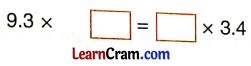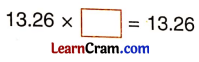# DAV Class 5 Maths Chapter 7 Worksheet 4 Solutions

The DAV Maths Book Class 5 Solutions and DAV Class 5 Maths Chapter 7 Worksheet 4 Solutions of Multiplication and Division of Decimal Numbers offer comprehensive answers to textbook questions.

## DAV Class 5 Maths Ch 7 Worksheet 4 Solutions

Question 1.
Complete the following:
(a)Solution:
5.8 × 6 = 6 × 5.8

(b)Solution:
0.8 × 0 = 0(c)Solution:
9.3 × 3.4 = 9.3 × 3.4

(d)Solution:
13.26 × 1 = 13.26

(e)Solution:
1 × 15.6 = 15.6

(f)Solution:
4.7 × 1 = 4.7(g)Solution:
15.5 × 1.8 = 15.5

(h)Solution:
95.601 × 0 = 0

DAV Class 5 Maths Chapter 7 Worksheet 4 Notes

If two decimal numbers are multiplied in either order, the product remains the same.
3.2 × 1.1 = 3.52
1.1 × 3.2 = 3.52
The product remains the same.The product of a decimal number and one is the decimal number itself.
7.32 × 1 = 7.32
18.86 × 1 = 18.56

The product of a decimal number and zero is always zero.
5.78 × 0 = 0
18.96 × 0 = 0